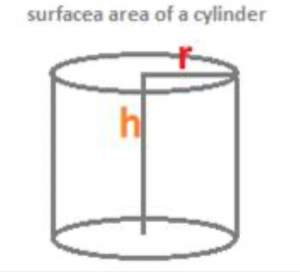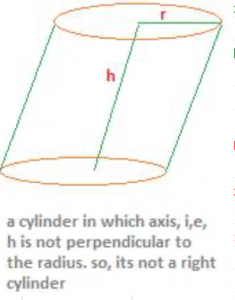Note:

1. In this page on the surface area of a cylinder, the word cylinder always refers to a right circular cylinder.
2. Now, the surface area of a cylinder can mean either curved surface area of a cylinder (i.e. a right circular cylinder) or the total surface area of a cylinder.
3. Curved surface area of a cylinder is the round surface without including the two circular planes at the two ends of the cylinder.
4. Total surface area of a cylinder is the sum of the curved surface area of a cylinder and the two closed circular faces at the two ends of the cylinder.

A cylinder in which the axis of the cylinder is perpendicular to the radius is called a right circular cylinder. The axis of a right circular cylinder is the line perpendicular to the radii of the two circular planes at the two ends. It is also called the height of a right circular cylinder. The height of a right circular cylinder is also called as length of the cylinder. A cylinder in which the axis is not perpendicular to the radii is not called a right circular cylinder. It is just a circular cylinder, if the two end-planes are circular in shape.And again, a cylinder in which the two ends are not circular planes is called a cylinder merely, not a circular cylinder.

How a right circular cylinder is formed?

Consider a paper, rectangular in shape. Let its dimensions, i.e. the length and breadth be l and hrespectively. Imagine the rectangular piece of paper rolled over its length. Then, a smooth, curved surface is formed. This new shape of the paper is a right circular cylinder. It is a right cylinder, because the line of rotation of the paper, which the breadth of the rectangle is, is perpendicular to the circular base of the cylinder, which is formed along the length of the rectangular piece of paper. Now, the area of the paper (rectangular in shape) must be equal to the curved surface area of the cylinder formed. Area of the rectangular piece of paper = l × h but, length of the paper = circular base of the cylinder

Therefore, l = 2Πr.

Finally, the curved surface area of a cylinder = 2Πr× h = 2Πrh.

The line of rotation is called the axis of the cylinder which is also called as the height or the length of the cylinder. In the formula 2Πrh, for the curved surface area of a cylinder, h stands for this height or length of the right circular cylinder.

Total surface area of a cylinder:

The total surface area of a cylinder is the sum of the curved surface area of a cylinder and the two closed circular faces at the two ends of the cylinder. A right circular cylinder with closed circular ends at the two ends will have total surface area too, besides the curved surface area.

Curved surface area of a cylinder = 2Πrh

Area of the two closed circular ends = Πr2 + Πr2 = 2Πr2

Therefore, the total surface area of a right circular cylinder = 2Πrh + 2Πr2

Curved surface area of a cylinder = 2Πrh, and

Total surface area of a cylinder = 2Πrh + 2Πr2